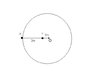# Circular motion

Rowshan
[SOLVED] Circular motion

## Homework Statement

two spheres of equal mass are attached to a light wire of negligible mass of length 3 m ,as shown in the diagram attatched, the spheres and the rod are whirled about point O at a constant rate.

## Homework Equations

F=ma
a= 4pi^2r/T^2

Find:
(the tension in the wire between spheres X and Y)/(Tension in the wire between sphere Y and O)

## The Attempt at a Solution

Finding the "Tension in the wire between sphere Y and O":

acceleration = (4pi^2(1))/(T^2) since r=1m

From f=ma
there are two masses flying around so ill let the mass equal to 2m.

therefore F= 2m x (4pi^2)/t^2

I don't know how to approach the rest of the problem. I don't even know if I've done it correctly so far.

#### Attachments

•Physics.JPG
5.9 KB · Views: 334

Homework Helper
You know the acceleration of each mass from your formula. The force acting on the mass X is the tension in the wire between X and Y. The force acting on Y is the difference in the tension between X and Y and that between Y and O (since they pull in opposite ways on Y). Now use F=ma. What must the ratio of the tensions be to give you the ratio of the accelerations you need?

Rowshan
You know the acceleration of each mass from your formula. The force acting on the mass X is the tension in the wire between X and Y. The force acting on Y is the difference in the tension between X and Y and that between Y and O (since they pull in opposite ways on Y). Now use F=ma. What must the ratio of the tensions be to give you the ratio of the accelerations you need?

Im confused. How can they pull opposite ways on Y. I thought there was no such thing as centrifugal force?

Homework Helper
Who said there was a centrifugal force? I was talking about the tension forces. They are real forces, and the accelerations are real accelerations. Use F=ma. No fictitious forces here. What's the net force acting on X and what's the net force acting on Y?

Last edited:
Rowshan
Ok But for f=ma should i use 2m for Y and just 1m for X? Why are the pulling opposite ways?

Rowshan
Ok But for f=ma should i use 2m for Y and just 1m for X? Why are Y being pulled opposite ways?

Homework Helper
The sphere are of equal mass, use m for both. A tension along a wire pulls the body it's attached to in the direction of the wire. Y has two wires attached to it. One connects it to the center, the other connects it to the outer mass. They are pulling it in different directions. Call TXY the tension in the XY part and TOY the tension in the OY part. In terms of those, what's the force on X and what's the force on Y?

Rowshan
I really need help visualising it. Is it like this?

X---->------<--Y-->--O

TOY=m*a

TXY+TOY=ma + m*3a
=4ma
therefore TOY/(TXY+TOY)=.25?

but that's not the answer in the back. the answer is .75?
Is this question supposed to be so difficult?
i have an year 12 phys exam on wednesday- I'm totally screwed.

Homework Helper
Don't panic. You have some of this right. Besides Wednesday is a long ways away. Yes, that's the picture. You have to consider the two masses separately. aX=TXY/m. aY=(TOY-TXY)/m. As you said aX=3*aY. Can you put that all together to find TXY/TOY=3/4?

Rowshan
Im sorry i still don't understand the direction of these tension forces

why is it like this

X-------->----<--------Y-->--O

ANd not also like this

x---->---<----Y--->--<---O

why is there forces acting this way <---- its this a action/reaction pair or something?

Rowshan
TXY = m*3a
TOY = ma+m*3a

TXY/TOY = 3/4
IS this right?
Even if it is still don't understand how or why. the tension forces are confusing me. Thnks a lot.

Rowshan
Okay could you please check if my reasoning is right:

FXY=Fnet on XY

FNet on FXY= m*3a

FXY=m*3a
--->

There is a reaction force on Mass Y so it suffers FXY in the opposite direction:
-m*3a
<---

Fnet on Mass Y is F=ma
Ma
->

FOY is the tension

FOY +(-FXY) = Fnet
so we have
FOY +-m*3a = ma
FOY + <--- = ->

Therefore
FOY = 4ma
FOY = -(<---) + ->
= ---->

Hence FXY/FOY = 3/4